Next: Infinite body with circular Up: Cylindrical Coordinates. Transient 1-D. Previous: Cylindrical Coordinates. Transient 1-D.

### Infinite body, cylindrical coordinate, transient 1-D.

R00 Infinite region, 0 < r <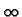.

 GR00(r, t | r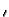,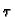) =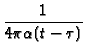exp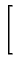-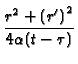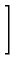I0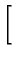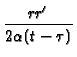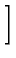Equivalent forms:
 GR00(r, t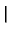r,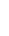) =exp-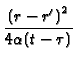x exp-I0GR00(r, tr,) =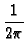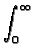exp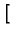-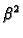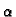(t -)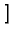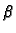J0(r) J0(r)dNote thatin the above integral has units of meters-1.

Special case when heat source is located at r'=0:

 GR00(r, t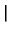0,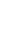) =exp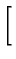-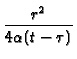Kevin D. Cole
2002-12-31